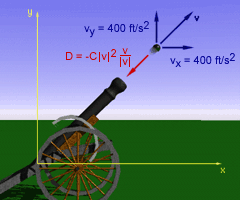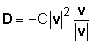Ch 2. Particle Force and Acceleration Multimedia Engineering Dynamics Rect.Coord. Normal/Tang. Coord. PolarCoord. Orbital Mechanics Computational Mechanics
 Chapter - Particle - 1. General Motion 2. Force & Accel. 3. Energy 4. Momentum - Rigid Body - 5. General Motion 6. Force & Accel. 7. Energy 8. Momentum 9. 3-D Motion 10. Vibrations Appendix Basic Math Units Basic Equations Sections Search eBooks Dynamics Fluids Math Mechanics Statics Thermodynamics Author(s): Kurt Gramoll ©Kurt GramollDYNAMICS - CASE STUDY IntroductionProblem Diagram A projectile is fired from a cannon into the air. The initial velocity of the projectile is known. The aerodynamic drag on the projectile is a function of the velocity. Integrating to find a solution for this problem could be difficult... Is there another way? What is known: The projectile is launched from x = 0, y = 0. The initial velocity of the projectile is vx = 400 ft/s       vy = 400 ft/s The aerodynamic drag force is given byQuestion What is the trajectory of the projectile for C = 0.002 ? C = 0.004 ? C = 0.006 ? Approach Because the force (and thus the acceleration) of the particle is given in terms of the absolute value of the velocity, integration to find position and velocity could be difficult. Solve this problem using a method of numeric integration, such as the finite-difference method. Drag equation is a vector relationship that needs to be applied in both vertical and horizontal direction.

Practice Homework and Test problems now available in the 'Eng Dynamics' mobile app
Includes over 400 problems with complete detailed solutions.
Available now at the Google Play Store and Apple App Store.